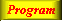Lecture 3 - Page 22 : 42
 Functional Programming in SchemeName binding, Recursion, Iteration, and Continuations * Name binding constructs The let name binding expression The equivalent meaning of let Examples with let name binding The let* name binding construct An example with let* The letrec namebinding construct LAML time functions * Conditional expressions Conditional expressions Examples with if Example with cond: leap-year? Example with cond: american-time Example with cond: as-string * Recursion and iteration Recursion List processing Tree processing (1) Tree processing (2) Recursion versus iteration Example of recursion: number-interval Examples of recursion: string-merge Examples with recursion: string-of-char-list? Exercises * Example of recursion: Hilbert Curves Hilbert Curves Building Hilbert Curves of order 1 Building Hilbert Curves of order 2 Building Hilbert Curves of order 3 Building Hilbert Curves of order 4 A program making Hilbert Curves * Continuations Introduction and motivation The catch and throw idea A catch and throw example The intuition behind continuations Being more precise The capturing of continuations Capturing, storing, and applying continuations Use of continuations for escaping purposes Practical example: Length of an improper list Practical example: Searching a binary tree
 Examples of recursion: string-merge
 The function string-merge zips two lists of strings to a single string. The lists are not necessarily of equal lengths
 ```(define (string-merge str-list-1 str-list-2) (cond ((null? str-list-1) (apply string-append str-list-2)) ((null? str-list-2) (apply string-append str-list-1)) (else (string-append (car str-list-1) (car str-list-2) (string-merge (cdr str-list-1) (cdr str-list-2))))))```

The recursive function string-merge. Notice that this function is a general recursive function. The recursive call, emphasized above, is not in a tail position, because of the embedding in string-append.An application of string-merge which converts a list of strings to a string with a given separator.

This is a typical task in a web program, where a list of elements needs to be aggregated for HTML presentation purposes. Notice the merging of a list of n elements with a list of length n-1. The function make-list is another LAML function; (make-list n el) makes a list of n occurrences of el.A tail recursive version of string-merge.